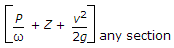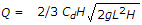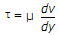# Civil Engineering - Hydraulics

### Exercise :: Hydraulics - Section 1

26.

While applying the Bernoulli's equation= total head, the work any section done on the flow system, if any

 A. is added on the right side of the equation B. is added on the left side of the equation C. is ignored D. none of these.

Answer: Option B

Explanation:

No answer description available for this question. Let us discuss.

27.

Discharge Q over a rectangular weir of length L and height H, is given by the equation

 A. Q = 2/3 Cd2g LH3/2 B. Q = 2/3 CdL H2gH C.D. all the above.

Answer: Option D

Explanation:

No answer description available for this question. Let us discuss.

28.

A short tube mouthpiece will not run full at its outlet if the head under which the orifice works, is

 A. less than 12.2 m of the water B. more than 12.2 m of the water C. equal of 12.2 m of water D. none of these.

Answer: Option B

Explanation:

No answer description available for this question. Let us discuss.

29.

Hydrostatic pressure on a dam depends upon its

 A. length B. depth C. shape D. material E. both (b) and (c).

Answer: Option E

Explanation:

No answer description available for this question. Let us discuss.

30.

When two layers of a fluid separated by dy move over the other with a difference of velocity dv, causes a shearing stress, where μ is known as

 A. coefficient of viscosity B. absolute viscosity C. dynamic viscosity D. viscosity E. all the above.

Answer: Option E

Explanation:

No answer description available for this question. Let us discuss.

#### Current Affairs 2021

Interview Questions and Answers